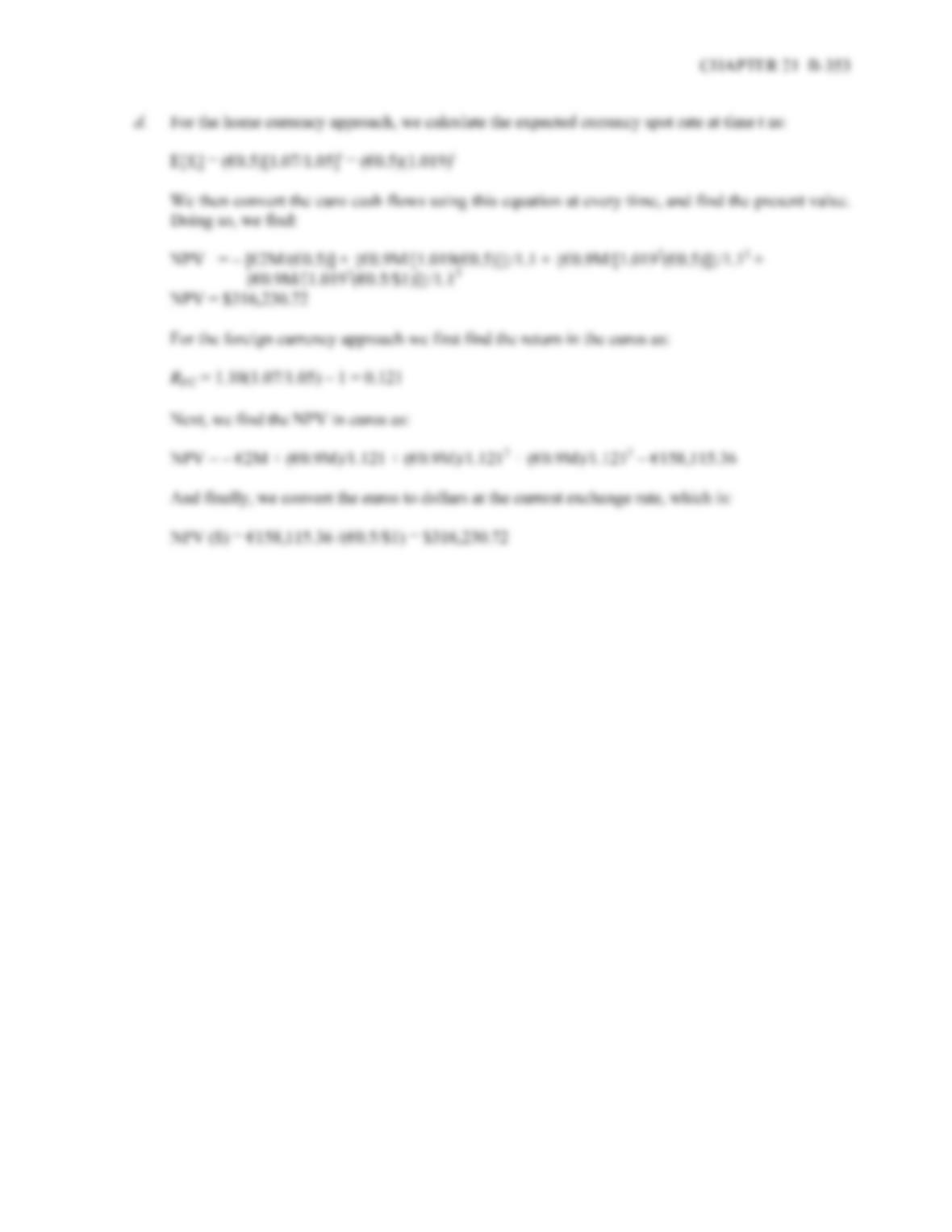Type
Quiz
Book Title
Fundamentals of Corporate Finance Standard Edition 9th Edition
ISBN 13
978-0073382395

### 978-0073382395 Chapter 21 Questions and Problems

April 3, 2019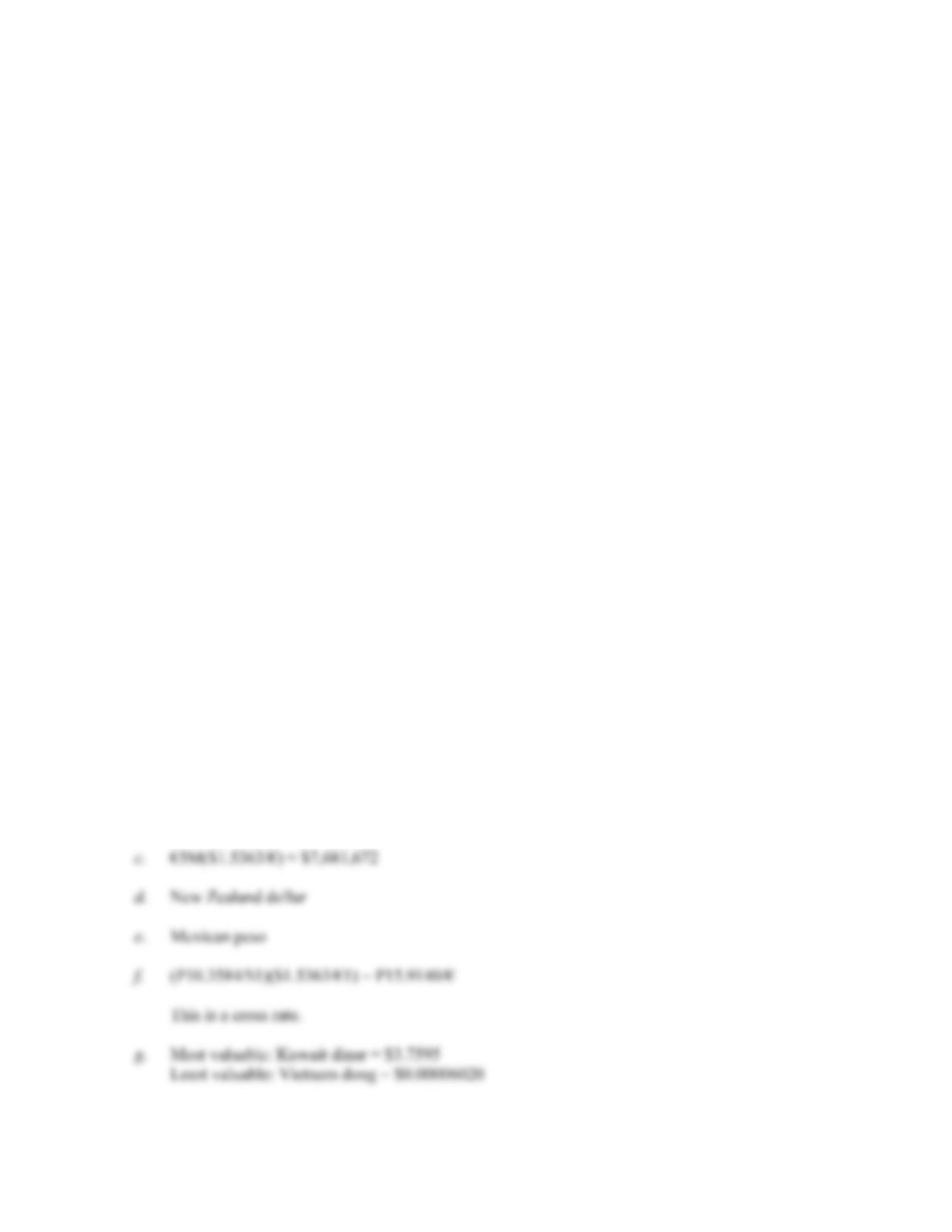CHAPTER 21 B-345
c. True. The market would only be correct on average, while you would be correct all the time.
9. a. American exporters: their situation in general improves because a sale of the exported goods for a
fixed number of euros will be worth more dollars.
American importers: their situation in general worsens because the purchase of the imported goods
for a fixed number of euros will cost more in dollars.
b. American exporters: they would generally be better off if the British government’s intentions result
in a strengthened pound.
American importers: they would generally be worse off if the pound strengthens.
c. American exporters: would generally be much worse off, because an extreme case of fiscal
expansion like this one will make American goods prohibitively expensive to buy, or else Brazilian
sales, if fixed in reais, would become worth an unacceptably low number of dollars.
American importers: would generally be much better off, because Brazilian goods will become
much cheaper to purchase in dollars.
10. IRP is the most likely to hold because it presents the easiest and least costly means to exploit any
arbitrage opportunities. Relative PPP is least likely to hold since it depends on the absence of market
imperfections and frictions in order to hold strictly.
SolutionstoQuestionsandProblems
NOTE: All end of chapter problems were solved using a spreadsheet. Many problems require multiple steps.
Due to space and readability constraints, when these intermediate steps are included in this solutions
manual, rounding may appear to have occurred. However, the final answer for each problem is found
without rounding during any step in the problem.
Basic
1. Using the quotes from the table, we get:
a. \$100(€0.6509/\$1) = €65.09
b. \$1.5363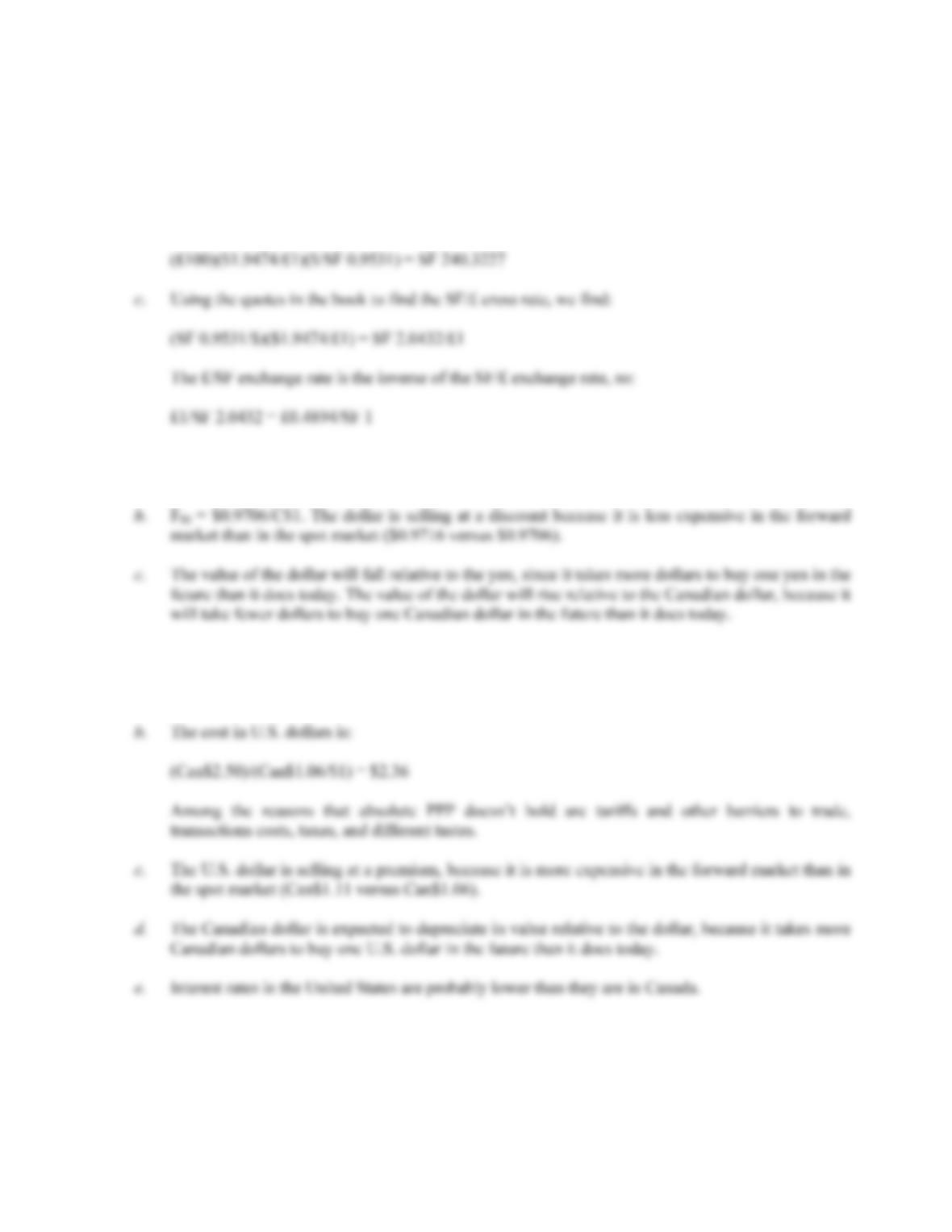B-346 SOLUTIONS
2. a. You would prefer £100, since:
(£100)(£1/\$0.5135) = \$194.74
b. You would still prefer £100. Using the \$/£ exchange rate and the SF/£ exchange rate to find the
amount of Swiss francs £100 will buy, we get:
3. a. F
180 = ¥106.96 (per \$). The yen is selling at a premium because it is more expensive in the forward
market than in the spot market (\$0.009241 versus \$0.0093493).
b. F
(Can\$1)/(Can\$1.06/\$1) = \$0.9434
5. a. The cross rate in ¥/£ terms is:
(¥112/\$1)(\$1.93/£1) = ¥216.16/£1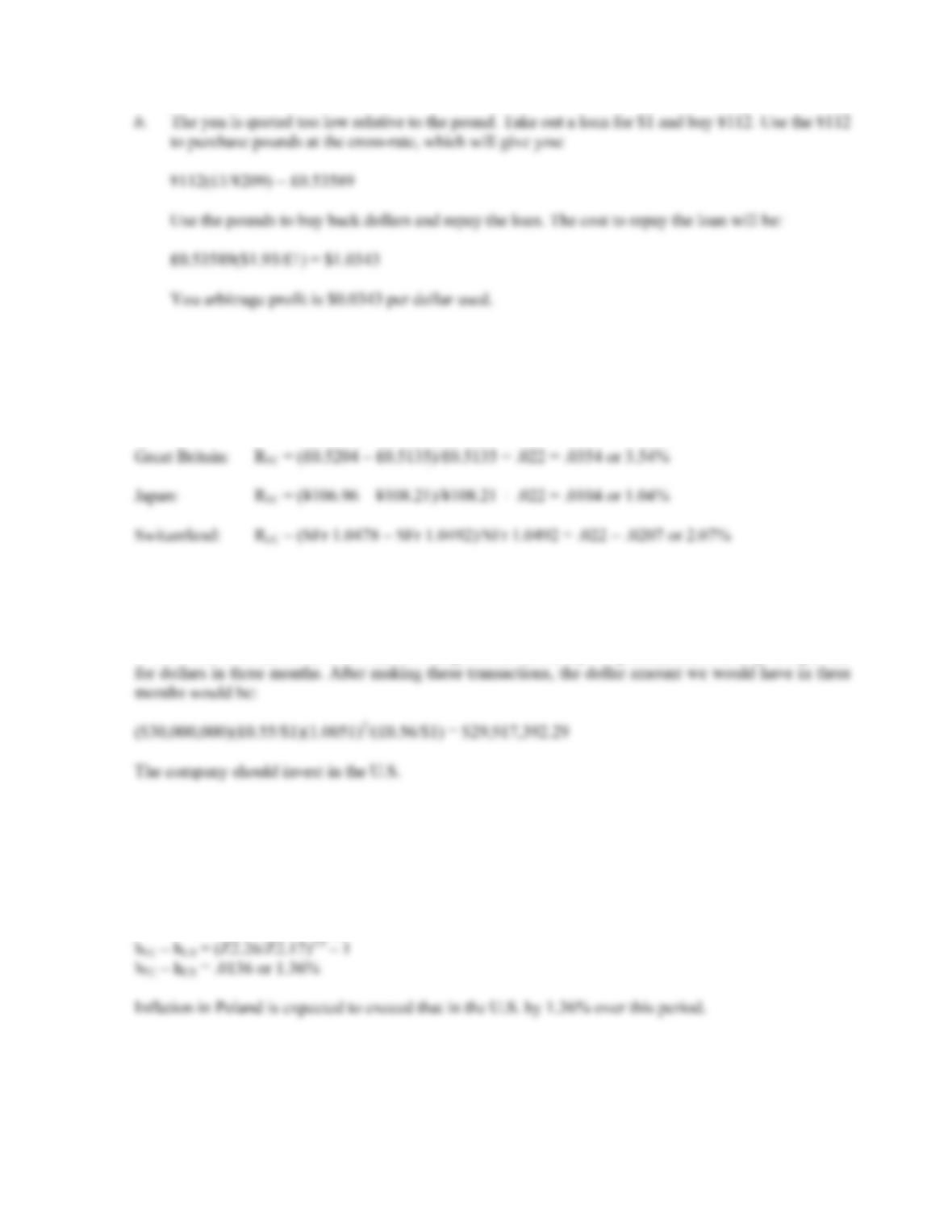CHAPTER 21 B-347
6. We can rearrange the interest rate parity condition to answer this question. The equation we will use is:
R
FC = (Ft – S0)/S0 + RUS
Using this relationship, we find:
7. If we invest in the U.S. for the next three months, we will have:
\$30,000,000(1.0037)3 = \$30,334,233.62
If we invest in Great Britain, we must exchange the dollars today for pounds, and exchange the pounds
8. Using the relative purchasing power parity equation:
F
t = S0 × [1 + (hFC – hUS)]t
We find:
Z2.26 = Z2.17[1 + (hFC – hUS)]3
h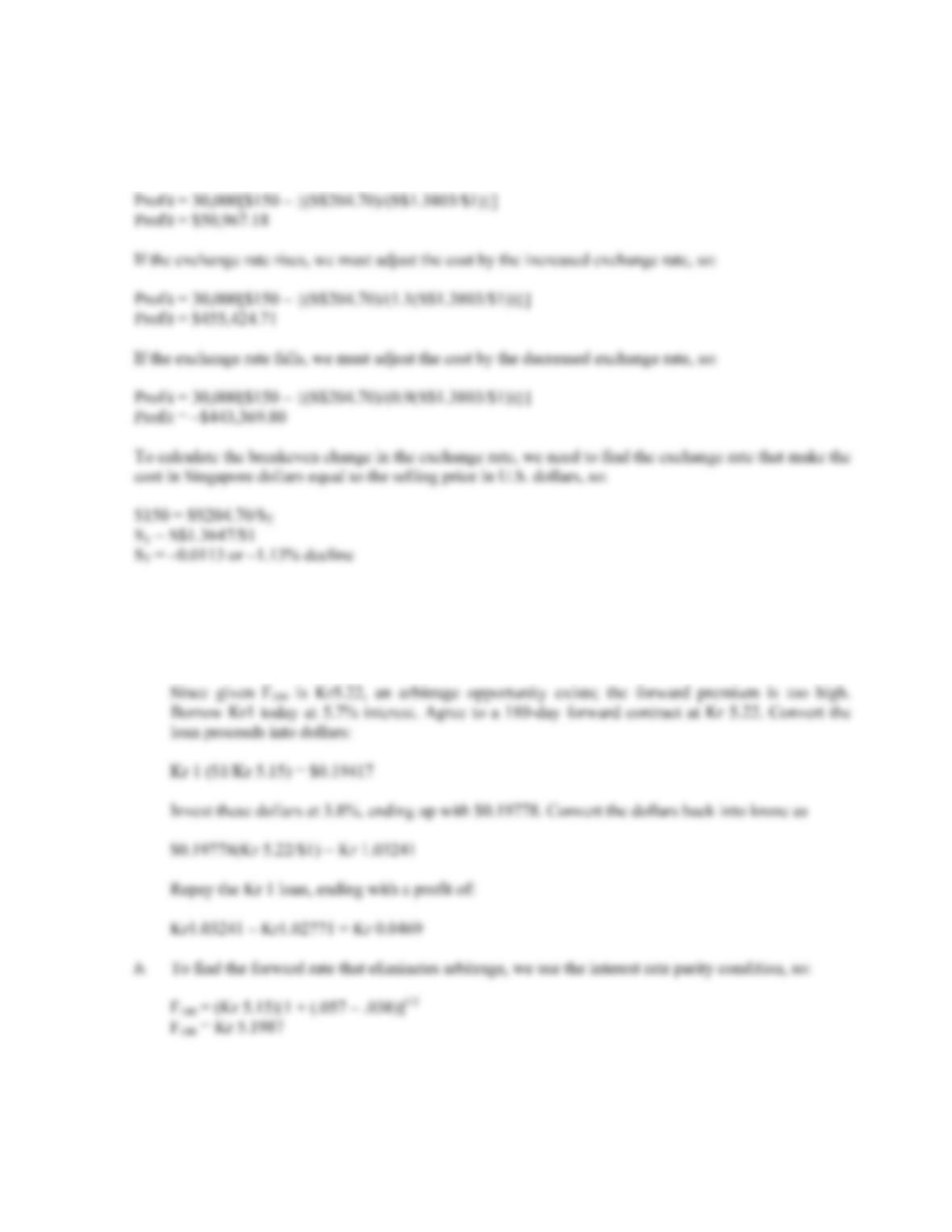B-348 SOLUTIONS
9. The profit will be the quantity sold, times the sales price minus the cost of production. The production
cost is in Singapore dollars, so we must convert this to U.S. dollars. Doing so, we find that if the
exchange rates stay the same, the profit will be:
10. a. If IRP holds, then:
F
180 = (Kr 5.15)[1 + (.057 – .038)]1/2
F
180 = Kr 5.1987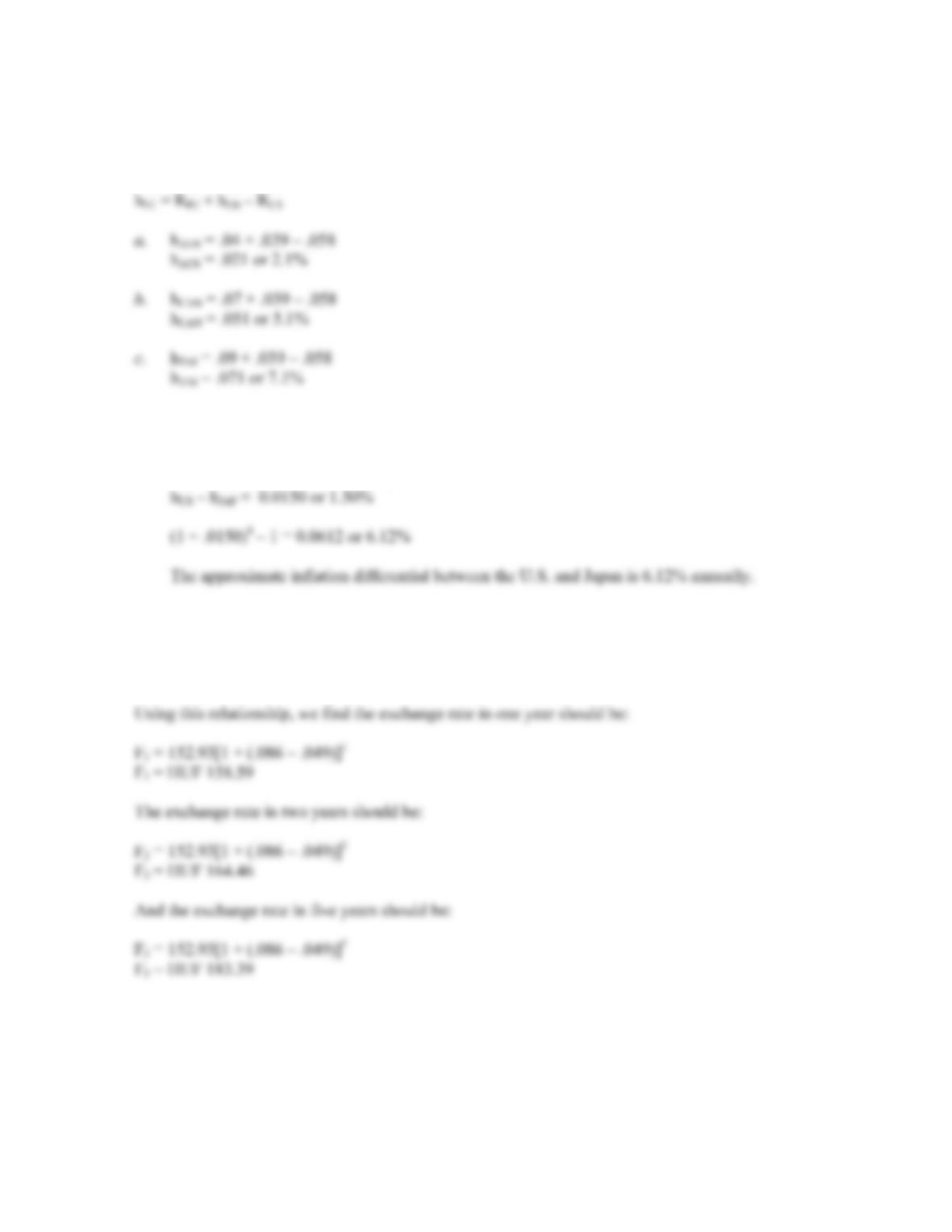CHAPTER 21 B-349
11. The international Fisher effect states that the real interest rate across countries is equal. We can
rearrange the international Fisher effect as follows to answer this question:
R
US – hUS = RFC – hFC
h
12. a. The yen is expected to get weaker, since it will take more yen to buy one dollar in the future than it
does today.
b. h
US – hJAP (¥116.03 – ¥114.32)/¥114.32
h
13. We need to find the change in the exchange rate over time so we need to use the interest rate parity
relationship:
F
t = S0 × [1 + (RFC – RUS)]t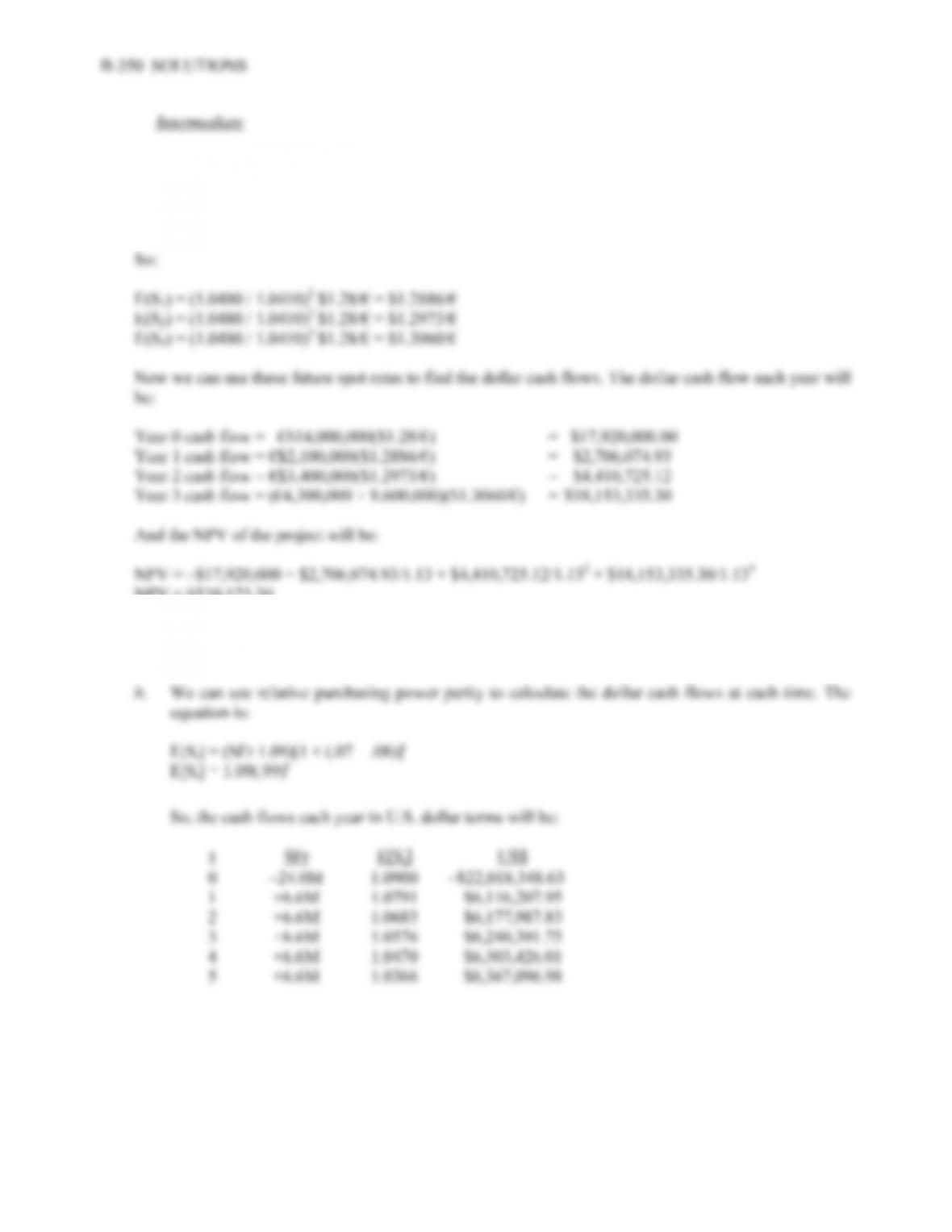14. First, we need to forecast the future spot rate for each of the next three years. From interest rate and
purchasing power parity, the expected exchange rate is:
E(ST) = [(1 + RUS) / (1 + RFC)]T S0
NPV = \$510,173.30
15. a. Implicitly, it is assumed that interest rates won’t change over the life of the project, but the
exchange rate is projected to decline because the Euroswiss rate is lower than the Eurodollar rate.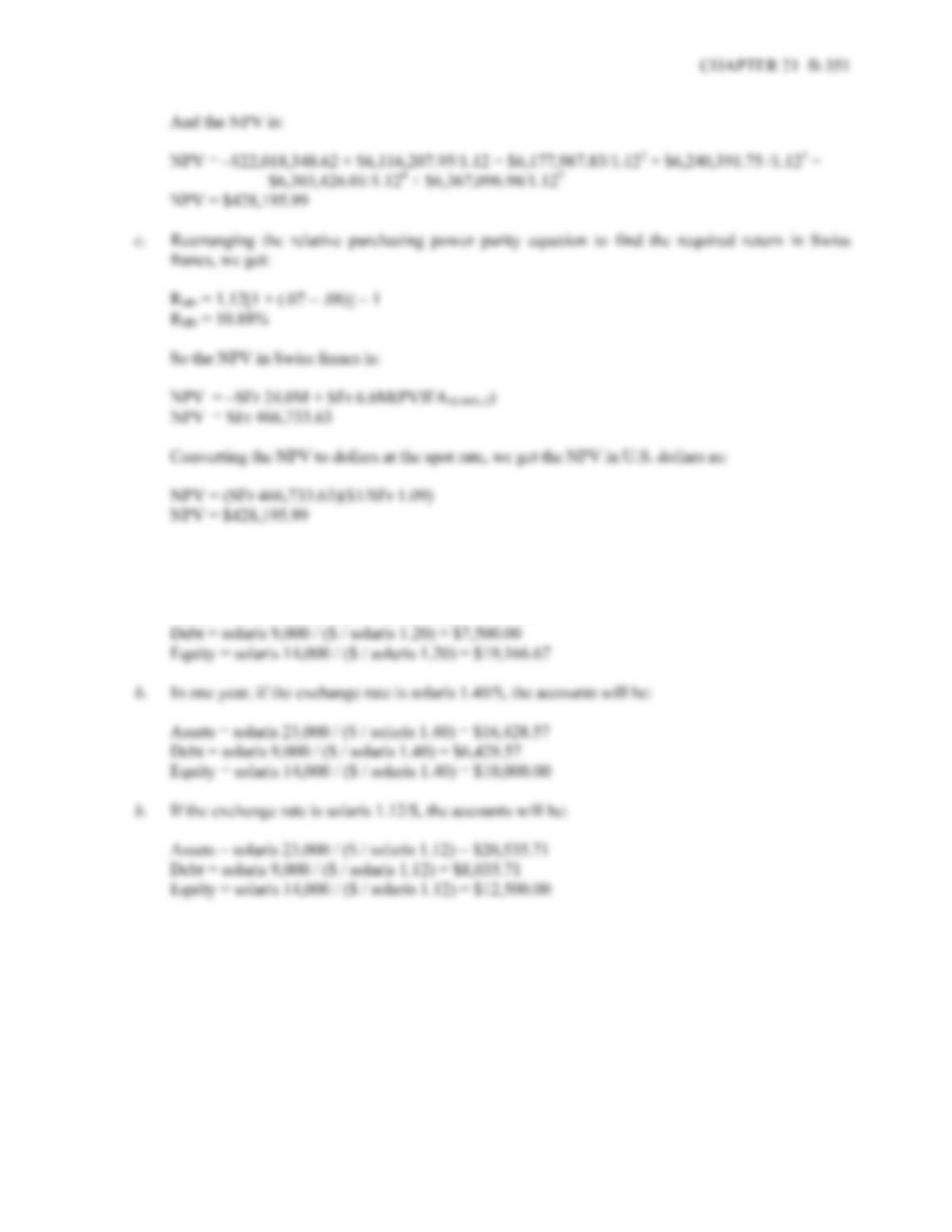16. a. To construct the balance sheet in dollars, we need to convert the account balances to dollars. At the
current exchange rate, we get:
Assets = solaris 23,000 / (\$ / solaris 1.20) = \$19,166.67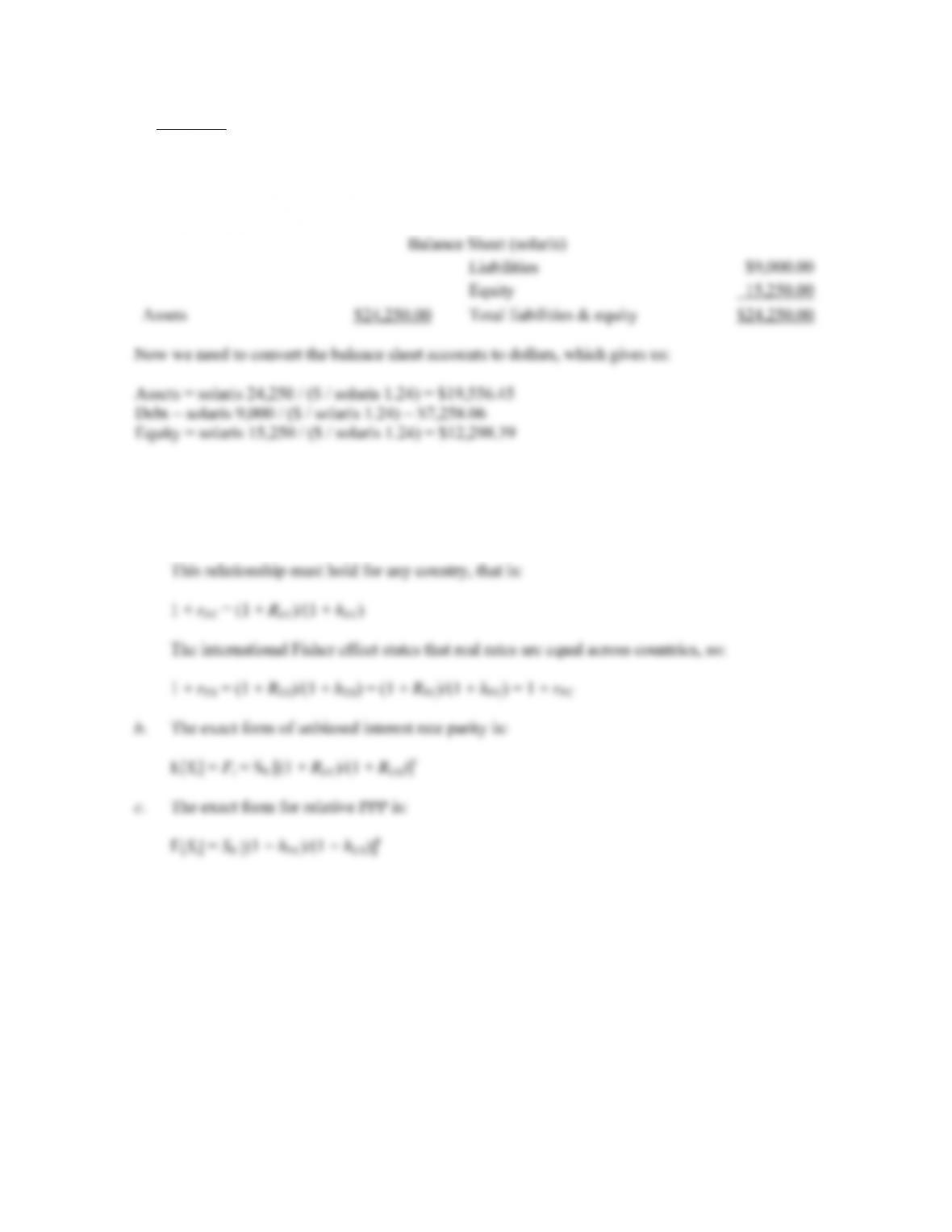B-352 SOLUTIONS
Challenge
17. First, we need to construct the end of year balance sheet in solaris. Since the company has retained
earnings, the equity account will increase, which necessarily implies the assets will also increase by the
same amount. So, the balance sheet at the end of the year in solaris will be:
18. a. The domestic Fisher effect is:
1 +
RUS = (1 + rUS)(1 + hUS)
1 +
rUS = (1 + RUS)/(1 + hUS)